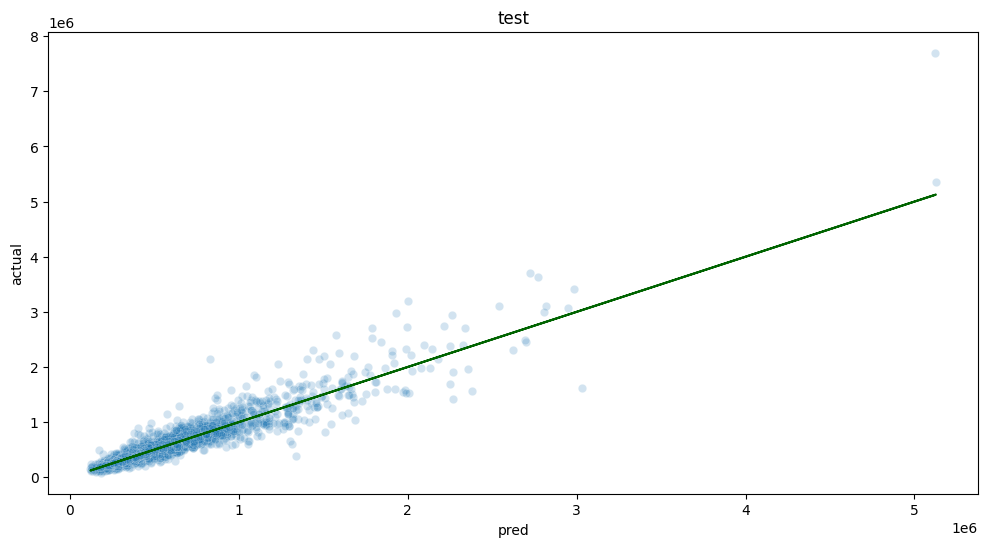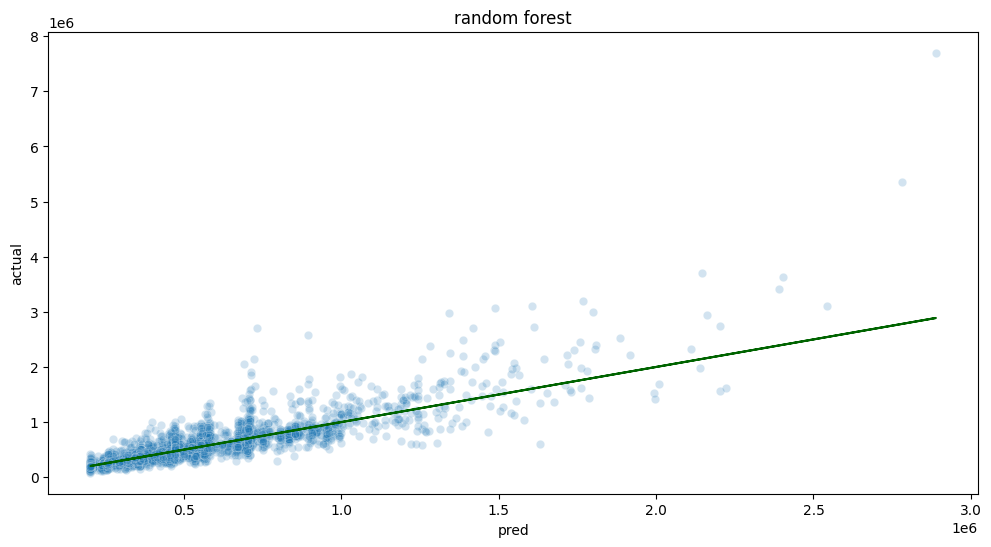## Data Exploration And Model Selection

The following tutorials are available from the Wallaroo Tutorials Repository.

# Stage 1: Data Exploration And Model Selection

When starting a project, the data scientist focuses on exploration and experimentation, rather than turning the process into an immediate production system. This notebook presents a simplified view of this stage.

## Resources

The following resources are used as part of this tutorial:

• data
• data/seattle_housing_col_description.txt: Describes the columns used as part data analysis.
• data/seattle_housing.csv: Sample data of the Seattle, Washington housing market between 2014 and 2015.
• code
• postprocess.py: Formats the data after inference by the model is complete.
• preprocess.py: Formats the incoming data for the model.
• simdb.py: A simulated database to demonstrate sending and receiving queries.
• wallaroo_client.py: Additional methods used with the Wallaroo instance to create workspaces, etc.

## Steps

The following steps are part of this process:

### Import Libraries

First we’ll import the libraries we’ll be using to evaluate the data and test different models.

import numpy as np
import pandas as pd

import sklearn
import sklearn.ensemble

import xgboost as xgb

import seaborn
import matplotlib
import matplotlib.pyplot as plt

import simdb # module for the purpose of this demo to simulate pulling data from a database

matplotlib.rcParams["figure.figsize"] = (12,6)

# ignoring warnings for demonstration
import warnings
warnings.filterwarnings('ignore')


### Retrieve Training Data

For training, we will use the data on all houses sold in this market with the last two years. As a reminder, this data pulled from a simulated database as an example of how to pull from an existing data store.

Only a few columns will be shown for display purposes.

conn = simdb.simulate_db_connection()
tablename = simdb.tablename

query = f"select * from {tablename} where date > DATE(DATE(), '-24 month') AND sale_price is not NULL"
print(query)

conn.close()
housing_data.loc[:, ["id", "date", "list_price", "bedrooms", "bathrooms", "sqft_living", "sqft_lot"]]

select * from house_listings where date > DATE(DATE(), '-24 month') AND sale_price is not NULL

id date list_price bedrooms bathrooms sqft_living sqft_lot
0 7129300520 2022-10-05 221900.0 3 1.00 1180 5650
1 6414100192 2022-12-01 538000.0 3 2.25 2570 7242
2 5631500400 2023-02-17 180000.0 2 1.00 770 10000
3 2487200875 2022-12-01 604000.0 4 3.00 1960 5000
4 1954400510 2023-02-10 510000.0 3 2.00 1680 8080
... ... ... ... ... ... ... ...
20518 263000018 2022-05-13 360000.0 3 2.50 1530 1131
20519 6600060120 2023-02-15 400000.0 4 2.50 2310 5813
20520 1523300141 2022-06-15 402101.0 2 0.75 1020 1350
20521 291310100 2023-01-08 400000.0 3 2.50 1600 2388
20522 1523300157 2022-10-07 325000.0 2 0.75 1020 1076

20523 rows × 7 columns

### Data transformations

To improve relative error performance, we will predict on log10 of the sale price.

Predict on log10 price to try to improve relative error performance

housing_data['logprice'] = np.log10(housing_data.sale_price)


From the data, we will create the following features to evaluate:

• house_age: How old the house is.
• renovated: Whether the house has been renovated or not.
• yrs_since_reno: If the house has been renovated, how long has it been.
import datetime

thisyear = datetime.datetime.now().year

housing_data['house_age'] = thisyear - housing_data['yr_built']
housing_data['renovated'] =  np.where((housing_data['yr_renovated'] > 0), 1, 0)
housing_data['yrs_since_reno'] =  np.where(housing_data['renovated'], housing_data['yr_renovated'] - housing_data['yr_built'], 0)

housing_data.loc[:, ['yr_built', 'yr_renovated', 'house_age', 'renovated', 'yrs_since_reno']]

yr_built yr_renovated house_age renovated yrs_since_reno
0 1955 0 68 0 0
1 1951 1991 72 1 40
2 1933 0 90 0 0
3 1965 0 58 0 0
4 1987 0 36 0 0
... ... ... ... ... ...
20518 2009 0 14 0 0
20519 2014 0 9 0 0
20520 2009 0 14 0 0
20521 2004 0 19 0 0
20522 2008 0 15 0 0

20523 rows × 5 columns

Now we pick variables and split training data into training and holdout (test).

vars = ['bedrooms', 'bathrooms', 'sqft_living', 'sqft_lot', 'floors', 'waterfront', 'view',
'condition', 'grade', 'sqft_above', 'sqft_basement', 'lat', 'long', 'sqft_living15', 'sqft_lot15', 'house_age', 'renovated', 'yrs_since_reno']

outcome = 'logprice'

runif = np.random.default_rng(2206222).uniform(0, 1, housing_data.shape)
gp = np.where(runif < 0.2, 'test', 'training')

hd_train = housing_data.loc[gp=='training', :].reset_index(drop=True, inplace=False)
hd_test = housing_data.loc[gp=='test', :].reset_index(drop=True, inplace=False)

# split the training into training and val for xgboost
runif = np.random.default_rng(123).uniform(0, 1, hd_train.shape)
xgb_gp = np.where(runif < 0.2, 'val', 'train')

# for xgboost, further split into train and val
train_features = np.array(hd_train.loc[xgb_gp=='train', vars])
train_labels = np.array(hd_train.loc[xgb_gp=='train', outcome])

val_features = np.array(hd_train.loc[xgb_gp=='val', vars])
val_labels = np.array(hd_train.loc[xgb_gp=='val', outcome])


#### Postprocessing

Since we are fitting a model to predict log10 price, we need to convert predictions back into price units. We also want to round to the nearest dollar.

def postprocess(log10price):
return np.rint(np.power(10, log10price))


### Model testing

For the purposes of this demo, let’s say that we require a mean absolute percent error (MAPE) of 15% or less, and the we want to try a few models to decide which model we want to use.

One could also hyperparameter tune at this stage; for brevity, we’ll omit that in this demo.

#### XGBoost

First we will test out using a XGBoost model.


xgb_model = xgb.XGBRegressor(
objective = 'reg:squarederror',
max_depth=5,
base_score = np.mean(hd_train[outcome])
)

xgb_model.fit(
train_features,
train_labels,
eval_set=[(train_features, train_labels), (val_features, val_labels)],
verbose=False,
early_stopping_rounds=35
)

XGBRegressor(base_score=5.666446833601829, booster='gbtree', callbacks=None,
colsample_bylevel=1, colsample_bynode=1, colsample_bytree=1,
early_stopping_rounds=None, enable_categorical=False,
eval_metric=None, gamma=0, gpu_id=-1, grow_policy='depthwise',
importance_type=None, interaction_constraints='',
learning_rate=0.300000012, max_bin=256, max_cat_to_onehot=4,
max_delta_step=0, max_depth=5, max_leaves=0, min_child_weight=1,
missing=nan, monotone_constraints='()', n_estimators=100, n_jobs=0,
num_parallel_tree=1, predictor='auto', random_state=0, reg_alpha=0,
reg_lambda=1, ...)
In a Jupyter environment, please rerun this cell to show the HTML representation or trust the notebook.
XGBRegressor(base_score=5.666446833601829, booster='gbtree', callbacks=None,
         colsample_bylevel=1, colsample_bynode=1, colsample_bytree=1,
early_stopping_rounds=None, enable_categorical=False,
eval_metric=None, gamma=0, gpu_id=-1, grow_policy=&#x27;depthwise&#x27;,
importance_type=None, interaction_constraints=&#x27;&#x27;,
learning_rate=0.300000012, max_bin=256, max_cat_to_onehot=4,
max_delta_step=0, max_depth=5, max_leaves=0, min_child_weight=1,
missing=nan, monotone_constraints=&#x27;()&#x27;, n_estimators=100, n_jobs=0,
num_parallel_tree=1, predictor=&#x27;auto&#x27;, random_state=0, reg_alpha=0,
reg_lambda=1, ...)</pre>

print(xgb_model.best_score)
print(xgb_model.best_iteration)
print(xgb_model.best_ntree_limit)
0.07793614689092423
99
100

XGBoost Evaluate on holdout
With the sample model created, we will test it against the holdout data.  Note that we are calling the postprocess function on the data.
test_features = np.array(hd_test.loc[:, vars])
test_labels = np.array(hd_test.loc[:, outcome])

pframe = pd.DataFrame({
'pred' : postprocess(xgb_model.predict(test_features)),
'actual' : postprocess(test_labels)
})

ax = seaborn.scatterplot(
data=pframe,
x='pred',
y='actual',
alpha=0.2
)
matplotlib.pyplot.plot(pframe.pred, pframe.pred, color='DarkGreen')
matplotlib.pyplot.title("test")
plt.show()pframe['se'] = (pframe.pred - pframe.actual)**2

pframe['pct_err'] = 100*np.abs(pframe.pred - pframe.actual)/pframe.actual
pframe.describe()


pred
actual
se
pct_err

count
4.094000e+03
4.094000e+03
4.094000e+03
4094.000000

mean
5.340824e+05
5.396937e+05
1.657722e+10
12.857674

std
3.413714e+05
3.761666e+05
1.276017e+11
13.512028

min
1.216140e+05
8.200000e+04
1.000000e+00
0.000500

25%
3.167628e+05
3.200000e+05
3.245312e+08
4.252492

50%
4.568700e+05
4.500000e+05
1.602001e+09
9.101485

75%
6.310372e+05
6.355250e+05
6.575385e+09
17.041227

max
5.126706e+06
7.700000e+06
6.637466e+12
252.097895

rmse = np.sqrt(np.mean(pframe.se))
mape = np.mean(pframe.pct_err)

print(f'rmse = {rmse}, mape = {mape}')
rmse = 128752.54982046234, mape = 12.857674005250548

Random Forest
The next model to test is Random Forest.
model_rf = sklearn.ensemble.RandomForestRegressor(n_estimators=100, max_depth=5, n_jobs=2, max_samples=0.8)

train_features = np.array(hd_train.loc[:, vars])
train_labels = np.array(hd_train.loc[:, outcome])

model_rf.fit(train_features, train_labels)
RandomForestRegressor(max_depth=5, max_samples=0.8, n_jobs=2)In a Jupyter environment, please rerun this cell to show the HTML representation or trust the notebook. On GitHub, the HTML representation is unable to render, please try loading this page with nbviewer.org.RandomForestRegressorRandomForestRegressor(max_depth=5, max_samples=0.8, n_jobs=2)
Random Forest Evaluate on holdout
With the Random Forest sample model created, now we can test it against the holdout data.
pframe = pd.DataFrame({
'pred' : postprocess(model_rf.predict(test_features)),
'actual' : postprocess(test_labels)
})

ax = seaborn.scatterplot(
data=pframe,
x='pred',
y='actual',
alpha=0.2
)
matplotlib.pyplot.plot(pframe.pred, pframe.pred, color='DarkGreen')
matplotlib.pyplot.title("random forest")
plt.show()pframe['se'] = (pframe.pred - pframe.actual)**2

pframe['pct_err'] = 100*np.abs(pframe.pred - pframe.actual)/pframe.actual
pframe.describe()


pred
actual
se
pct_err

count
4.094000e+03
4.094000e+03
4.094000e+03
4094.000000

mean
5.194535e+05
5.396937e+05
3.875433e+10
18.188652

std
2.797001e+05
3.761666e+05
4.054895e+11
17.634478

min
2.039200e+05
8.200000e+04
1.444000e+03
0.014729

25%
3.291252e+05
3.200000e+05
6.686879e+08
6.156760

50%
4.621880e+05
4.500000e+05
3.321332e+09
13.148593

75%
5.851052e+05
6.355250e+05
1.367023e+10
24.630187

max
2.888692e+06
7.700000e+06
2.314868e+13
175.444819

rmse = np.sqrt(np.mean(pframe.se))
mape = np.mean(pframe.pct_err)

print(f'rmse = {rmse}, mape = {mape}')
rmse = 196861.19318381665, mape = 18.188652142429135

Final Decision
At this stage, we decide to go with the xgboost model, with the variables/settings above.
With this stage complete, we can move on to Stage 2: Training Process Automation Setup.


•